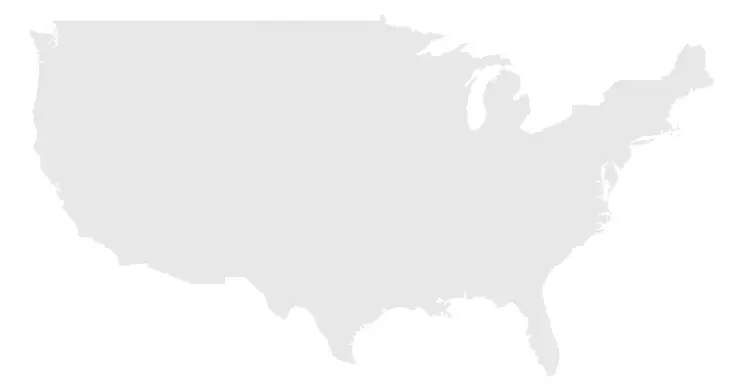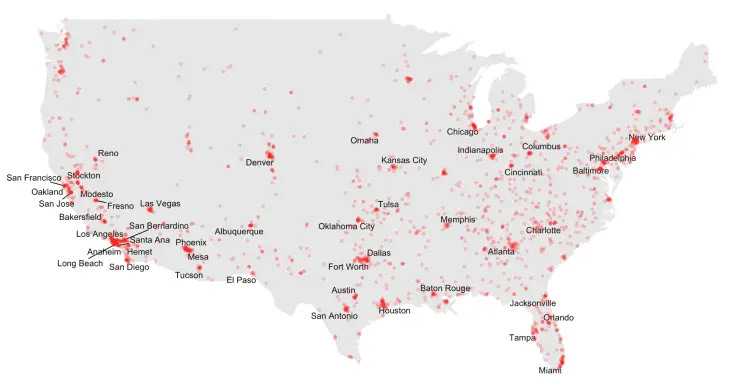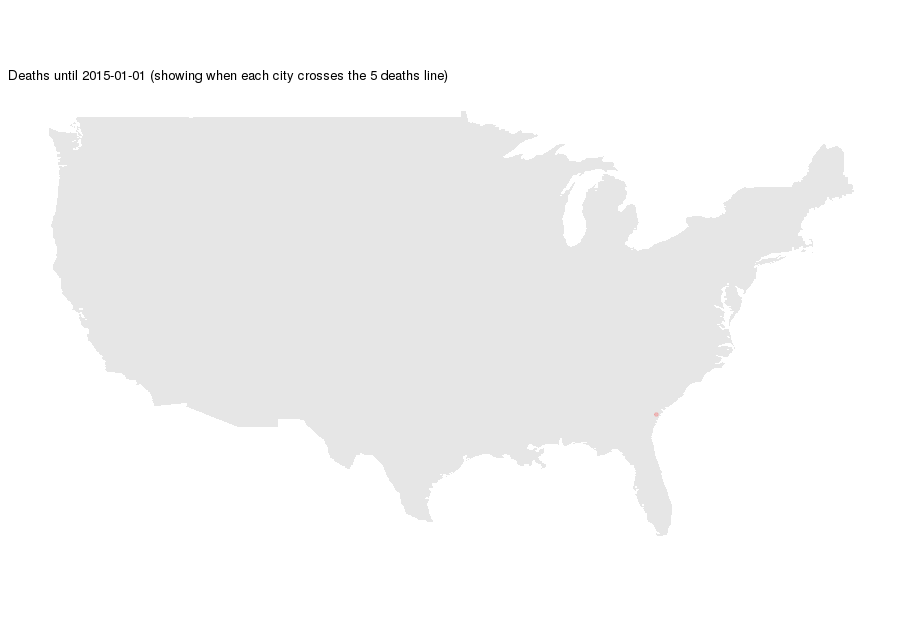# Space and time in R

While creating a Kaggle Kernel for the Killed by Police, 2015–2016 data set I had the idea to visualize the data as an animation. Since it contained information about every killing by police officer between 2015 and 2016 with the coordinates of each death, I figured each frame would be a plot that represented all deaths up to a particular day. It sounded very straightforward, but in the end it took more time than I expected.

## Creating the static plot

The first step was making a static plot so that I could have an idea of how I wanted the final product to look like. I had never plotted any geographic data with R before, so it was a while before I was able to get anything working. I decided to create a base layer for the plot and then worry about the data.

``````plot_deaths <- ggplot() +
geom_polygon(data = map_data("usa"), aes(long, lat, group = group), fill = "#e6e6e6") +
theme(axis.text.x = element_blank(), axis.text.y = element_blank(),
axis.title.x = element_blank(), axis.title.y = element_blank(),
axis.line = element_blank(), axis.ticks = element_blank(),
panel.background = element_blank(), panel.border = element_blank(),
panel.grid.major = element_blank(), panel.grid.minor = element_blank(),
legend.position = "none") +
coord_quickmap()
``````

The code above creates this image:Then I filtered the data set so that it only contained information about the continental US, which I called `cont_deaths`. After some minor tidying up, I also created a list of the cities (and their respective locations) that had more than 5 killings registered in the data set: `deadly_cities`.

``````plot_deaths +
geom_text_repel(data = deadly_cities, aes(long, lat, label = city), size = 4) +
geom_point(data = cont_deaths, aes(longitude, latitude), alpha = 0.2, color = "red") +
ggtitle("Killed by Police (showing cities with most deaths)")
``````

I tried using ggplot’s `geom_text` but many cities overlapped, so I went looking for a solution and ended up finding out about ggrepel. With `ggrepel::geom_text_repel`, the plot ended up looking pretty nice.

In the image below, the named cities are the ones with 5 or more deaths.I was very satisfied with the results, so I decided to start working on the animation.

## Creating the animation

To create the animation I used the animation package and installed ImageMagick. With `animation::saveGIF` all I had to do is loop through the plot I wanted for each frame and the rest was taken care of.

``````saveGIF(for (i in 0:730) {

# Filter deaths up to a certain date
time_deaths <- cont_deaths %>%
filter(date <= ymd("2015-01-01") + i)

# Get the cities that have already had more than 5 deaths
left_join(time_deaths, c("city" = "city", "country.etc" = "state")) %>%
group_by(city, country.etc) %>%
summarise(count = n(), long = long, lat = lat) %>%
ungroup() %>%
mutate(alph = count > 5)

# Plot deaths
print(plot_deaths +
geom_text_repel(data = time_cities, size = 4, segment.alpha = 0,
aes(long, lat, label = city, alpha = factor(alph))) +
scale_alpha_manual(values = c(0, 1)) +
geom_point(data = time_deaths, aes(longitude, latitude), alpha = 0.2, color = "red") +
ggtitle(paste0("Deaths until ", ymd("2015-01-01") + i,
" (showing when each city crosses the 5 deaths line)")))

}, "deaths.gif", interval = 0.005, ani.width = 900, ani.height = 630)
``````

In this snippet I loop through the 730 days of the data set and plot only the deaths up to that date. I also check to see if any city has crossed the 5 deaths line to start showing its name.

The final animation looks like this:## Final thoughts

Trying to create this animation was a very interesting experience. I had to look-up most of the stuff I was intending to do, but in the end I learned a lot. Special thanks to Rob Harrand, whose Kernel taught me to use the animation package.

The hardest part by far was getting the labels in the animation to behave. Since `ggrepel::geom_text_repel` finds the best spot for each label, as new cities crossed the 5 deaths mark, the other labels would jitter for some frames. I fixed this issue by having all of the labels be plotted right from the first frame, but leave the cities with 5 deaths or less with their alphas equal to zero.

If you want to take a look at the full source code, just head over to my Kernel. And if you want to take a look at other visualizations head over to my blog.